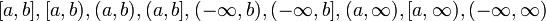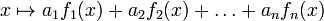# Difference between revisions of "Continuous functions form a vector space"

## Statement

### Continuity at a point version

Suppose$c \in \R$. Then, the following are true:

• Additive closure: If$f,g$ are functions defined in open intervals containing$c$ and both of them are continuous at$c$, then the pointwise sum$f + g$ is continuous at$c$.
• Scalar multiples: If$f$ is defined in an open interval containing$c$ and is continuous at$c$, and$\lambda$ is a real number, then$\lambda f$ is continuous at$c$.

There is a technical way of thinking of the collection of such functions as a real vector space, that basically involves identifying two functions as being the same function if they agree on an open interval containing$c$. This idea of identifying functions that look the same around$c$ is called taking the germ of a function and is beyond the scope of single variable calculus.

### Continuity around a point version

Suppose$c \in \R$. Then, the following are true:

• Additive closure: If$f,g$ are functions defined in open intervals containing$c$ and both of them are continuous on open intervals containing$c$, then the pointwise sum$f + g$ is continuous on an open interval containing$c$.
• Scalar multiples: If$f$ is defined and continuous in an open interval containing$c$ and is continuous at$c$, and$\lambda$ is a real number, then$\lambda f$ is continuous on an open interval containing$c$.

There is a technical way of thinking of the collection of such functions as a real vector space, that basically involves identifying two functions as being the same function if they agree on an open interval containing$c$. This idea of identifying functions that look the same around$c$ is called taking the germ of a function and is beyond the scope of single variable calculus.

### Continuity on an interval version

Suppose$I$ is an interval (possibly open, closed, or infinite from the left side and possibly open, closed, or infinite from the right side -- so it could be of the form$[a,b],[a,b),(a,b),(a,b],(-\infty,b),(-\infty,b],(a,\infty),[a,\infty),(-\infty,\infty)$). A continuous function on$I$ is a function on$I$ that is continuous at all points on the interior of$I$ and has the appropriate one-sided continuity at the boundary points (if they exist).

The continuous functions on$I$ form a real vector space, in the sense that the following hold:

• Additive closure: A sum of continuous functions is continuous: If$f,g$ are both continuous functions on$I$, so is$f + g$.
• Scalar multiples: If$\lambda \in \R$ and$f$ is a continuous function on$I$, then$\lambda f$ is also a continuous function on$I$.

We can also frame this in terms of linear combinations: if$f_1,f_2,\dots,f_n$ are all continuous functions and$a_1,a_2,\dots,a_n \in \R$, then the function$x \mapsto a_1f_1(x) + a_2f_2(x) + \dots + a_nf_n(x)$

is also a continuous function.

## Facts used

1. Limit is linear: This says that the limit of the sum is the sum of the limits, and scalar multiples can be pulled out of limits.